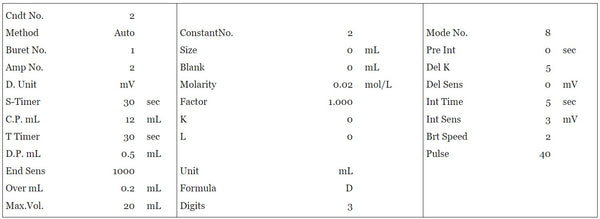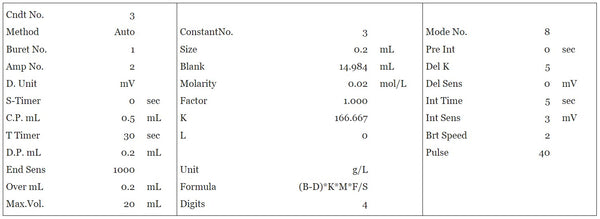HIRANUMA APPLICATION DATA Automatic Titrator Data No. E5 Feb. 10, 2021
 PLATING & ETCHING SOLUTION Quantitative determination of chromic anhydride and chromium (III) in chromium plating solution

## 1. Abstract

The control and analysis of chromium plating solution are very important process for the product quality. General analytical components of the chromium plating solution are the following 5 components.
(1) Chromic anhydride (CrO₃)
(2) Chromium (III) (Cr³⁺)
(3) Sulfuric acid
(4) Fluoride ion
(5) Iron
This report introduces an example of measurement for (1) chromic anhydride and (2) chromium (III).
The measurement procedure of chromic anhydride is as follows: Take diluted sample solution and add sulfuric acid to acidify it. Accurately add a certain amount of ammonium iron (II) sulfate in an amount sufficient to react the entire amount of chromic anhydride, and react the chromic anhydride by the reaction formula (1). The unreacted ammonium iron (II) sulfate is titrated with a potassium permanganate standard solution according to reaction formula (2) to obtain chromic anhydride.
The measurement procedure of chromium (III) is as follows: Take diluted sample solution and add sodium hydroxide to adjust it to pH 12 or more. Add hydrogen peroxide to oxidize chromium (III) to chromium (VI). Boil off and degrade the excess hydrogen peroxide by the reaction formula (3). Add sulfuric acid to acidify it. Accurately add a certain amount of ammonium iron (II) sulfate in an amount sufficient to react the entire amount of chromium (VI), and react the chromium (VI) by the reaction formula (1). The unreacted ammonium iron (II) sulfate is titrated with a potassium permanganate standard solution according to reaction formula (2) to obtain total chromium amount in term of chromic anhydride. Chromium (III) is obtained by subtracting chromic anhydride from total chromium amount.

 6Fe(NH₄)₂(SO₄)₂ ＋ 2CrO₃ ＋ 6H₂SO₄ → 3Fe₂(SO₄)₃ ＋ 6(NH₄)₂SO₄ ＋ Cr₂(SO₄)₃ + 6H₂O･･･(1) 2KMnO₄ + 10FeSO₄(NH₄)₂SO₄ ＋ 8H₂SO₄ → K₂SO₄ + 2MnSO₄ + 5Fe₂(SO₄)₃ ＋ 10(NH₄)₂SO₄+8H₂O･･･(2) 2[Cr(OH)₄]⁻ + 3H₂O₂ + 2OH⁻ → 2CrO₄²⁻ ＋ 8H₂O･･･(3)

## 2. Configuration of instruments and Reagents

 (1) Configuration of instruments Main unit : Automatic Titrator COM Series Main unit : Optional Buret 1 set Electrode : Platinum electrode PT-301 (Connect to IE-2)Reference electrode RE-201Z (Connect to RE-2) (2) Reagents Titrant : 0.02 mol/L potassium permanganate standard solution (For volumetric analysis) Additive solution : 10 % sodium hydroxide solution30 % hydrogen peroxide solution3 mol/L sulfuric acid0.1 mol/L ammonium iron (II) sulfate solutionDissolve 40 g of ammonium iron (II) sulfate hexahydrate in 30 mL of 10 % sulfuric acid solution, and pure water is added to make 1000 mL

## 3. Measurement procedure

3.1. Blank measurement

 (1) Dispense 90 mL of DI water and 10 mL of 3 mol/L sulfuric acid into a 200 mL beaker. (2) Immerse electrode and start measurement. Firstly dispense 15 mL of 0.1 mol/L ammonium iron (II) sulfate solution with optional buret No.2. (3) Titrate with 0.02 mol/L potassium permanganate standard solution. The obtained titrant volume is set to blank value of chromic anhydride and chromium (III) measurement.

3.2. Measurement of chromic anhydride

 (1) Take 1 mL of sample solution into a 50 mL volumetric flask and add DI water to make diluted sample solution. (2) Take 1 mL of diluted sample solution into a 200 mL beaker with volumetric pipette. Taking into account the dilution ratio, the net sample volume is 0.2 mL. (3) Add 90 mL of DI water and 10 mL of 3 mol/L sulfuric acid. (4) Immerse electrode and start measurement. Firstly dispense 15 mL of 0.1 mol/L ammonium iron (II) sulfate solution with optional buret No.2. Then titrate with 0.02 mol/L potassium permanganate standard solution.

3.3. Measurement of chromium (III)

 (1) Take 1 mL of diluted sample solution prepared on section 3.2. into a 200 mL beaker with volumetric pipette. Taking into account the dilution ratio, the net sample volume is 0.2 mL. (2) Add 40 mL of DI water and then add 10 % sodium hydroxide solution to adjust pH 12 or more. (3) Add 3 mL of 30 % hydrogen peroxide solution. (4) Add 100 mL of DI water and cover the beaker with watch glass. Heat on a hot plate until the amount of liquid becomes 100 mL or less. (5) After cooling to room temperature, rinse the inner wall of the watch glass and beaker with a small amount of pure water, and collect the rinse solution into the beaker. Add 10 mL of 3 mol/L sulfuric acid. (6) Immerse electrode and start measurement. Firstly dispense 15 mL of 0.1 mol/L ammonium iron (II) sulfate solution with optional buret No.2. Then titrate with 0.02 mol/L potassium permanganate standard solution.

## 4. Measurement conditions and results

#### Examples of titration conditions

(1) Blank measurement
Dispense of 0.1 mol/L ammonium iron (II) sulfate

 Cndt No. 1 Method Disp Buret No. 2 S-Timer 0 sec Disp.Vol. 15 mL

Titration with potassium permanganate(2) Measurement of chromic anhydride
Dispense of 0.1 mol/L ammonium iron (II) sulfate

 Cndt No. 1 Method Disp Buret No. 2 S-Timer 0 sec Disp.Vol. 15 mL

Titration with potassium permanganate(3) Measurement of chromium (III)
Dispense of 0.1 mol/L ammonium iron (II) sulfate

 Cndt No. 1 Method Disp Buret No. 2 S-Timer 0 sec Disp.Vol. 15 mL

Titration with potassium permanganateCalculation of chromium (III)### Measurement results

#### Result of blank

Number of
measurement
Titrant
volume(mL)
Statistic calculation
1 15.005 Avg. 14.984 mL
2 14.962 SD 0.030 mL
RSD 0.203 %

#### Example of titration curve#### Measurement results

##### Result of chromic anhydride
Number of
measurement
Sample
size
(mL)
Titrant
volume
(mL)
Chromic
anhydride
(g/L)
Statistic calculation
1 0.2 7.434 125.834 Avg. 126.13 g/L
2 0.2 7.398 126.434 SD 0.42 g/L
RSD 0.34 %

##### Example of titration curve#### Measurement results

##### Result of chromium (III)
Number of
measurement
Sample
size
(mL)
Titrant
volume
(mL)
Total
Chromium
(g/L as CrO3)
Chromium
(III)
(g/L as Cr(III))
Statistic calculation
1 0.2 7.238 129.100 1.5444 Avg. 1.61 g/L
2 0.2 7.223 129.350 1.6744 SD 0.09 g/L
RSD 5.71 %

##### Example of titration curve## 5. Note

 (1) Notice on pretreatmentIn the measurement of the total amount of chromium, if the residual hydrogen peroxide is not sufficiently removed by heating after the oxidation of chromium (III) to chromium (VI) by the hydrogen peroxide solution, a positive error will occur in the measurement. To prevent the measurement errors, it is important to perform the sufficient heat treatment to remove hydrogen peroxide. (2) Notice on back-titrationSince this measurement is performed by back titration, the accuracy of added ammonium iron (II) sulfate affects the measurement accuracy. In this paper, the accuracy is improved by automatic dispensing with burette. In addition, ammonium iron (II) sulfate is an unstable reagent that causes a decrease in concentration due to oxidation by air. It is important to check the blank value by performing blank measurement as appropriate.

 Keywords： Chromium plating solution, Chromic anhydride, Total chromium, Chromium (III), Chromium (VI)

Featured Instruments

View all